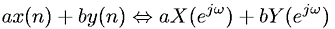Equations > Signal Processing > Fourier Transform Properties > Discrete-Time Fourier linearity theorem

### Discrete-Time Fourier linearity theoremLatex Code:

MathML Code:

 $\mathrm{ax}\left(n\right)+\mathrm{by}\left(n\right)⇔\mathrm{aX}\left({e}^{j\omega }\right)+\mathrm{bY}\left({e}^{j\omega }\right)$

MathType 5.0: